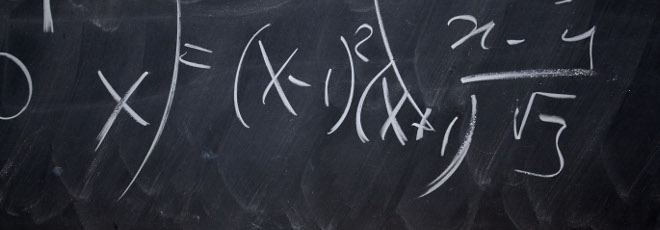## “Resolvent estimates inside the numerical range of non-self-adjoint operators and applications”

Martedì 17 Dicembre 2019, ore 12:20 - Aula Seminari 430 - Francesco Ferraresso (Università di Berna - Svizzera)

Abstract

Given a self-adjoint operator $T$ in a Hilbert space $\mathscr{H}$, it is well-known that $\left\lVert(T – \lambda)^{-1}\right\rVert = dist(\lambda, \sigma(T))^{-1}$ for all $\lambda$ in the resolvent set $\rho(T) = \mathbb{C} \,\backslash\sigma(T)$ of $T$.

Hence, the points $\lambda \in \mathbb{C}$ close to the spectrum of $T$ are exactly those having “big” $\left\lVert(T – \lambda)^{-1}\right\rVert$. However, this dramatically fails in the non-self-adjoint setting. Indeed, for a closed operator $T$ in $\mathscr{H}$ with $\overline{W(T)}\neq \mathbb{C}$, one only has $$\left\lVert(T – \lambda)^{-1}\right\rVert \le dist(\lambda, W(T))^{-1} \; \textrm{ for all } \lambda \in \mathbb {C} \, \backslash \overline{W(T)}\mathrm{,}$$ where $W(T)$ is the so-called numerical range of $T$. It follows that there may exist points $\lambda \in \rho(T) \cap\, \overline{W(T)}$ having a huge resolvent norm (the collection of such points is usually called pseudospectrum of $T$). Known examples show that the pseudospectrum of a closed operator $T$ with $\rho(T ) = \mathbb{C}$ may even cover the whole of $\mathbb{C}$.

The aim of this talk is to show that for particular classes of non-self-adjoint closed operators in Hilbert spaces it is possible to prove resolvent estimates in delimited areas located inside the numerical range. We will consider a class of $\mathcal{J}$-self-adjoint operator matrices $\mathscr{A}$ arising naturally in the study of systems of partial differential equations. While $W(\mathscr{A})$ is a parabolic region contained in the left complex half-plane with vertex in $0$, we will show that $\sigma(\mathscr{A})$ is real close to $0$. Furthermore, there exists $a \lt 0$ such that the strip $\{\lambda \in \mathbb{C}\,\backslash \mathbb{R} : 0 \gt \textrm{Re} \lambda \gt a\}$ admits a resolvent estimate in terms of the distance from the spectrum. Applications to Stokes-type systems will be briefly discussed.

This talk is based on an ongoing work with C. Tretter.